### Home > MC2 > Chapter 5 > Lesson 5.1.1 > Problem5-7

5-7.
1. Write the inverse operations, that is, operations that "undo" one another for each situation below. Homework Help ✎

1. What is the inverse operation for "add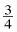?"

2. What is the inverse operation for "subtract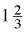?"

3. What is the inverse operation for "divide by 8?"

4. What is the inverse operation for "multiply by 12?"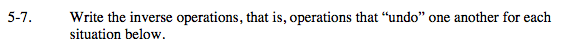Addition and subtraction are inverse operations.
For example, adding 4 and then subtracting 4 takes you back to your original number.

Multiplication and divsion are also inverse operations.
For example, multiplying a number by 4 and then dividing by 4 takes you back to your original number.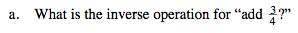$\text{Subtract}\ \frac{3}{4}.$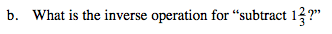If subtraction and addition are inverse operations, how much do you have to add?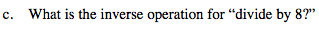Multiply by 8.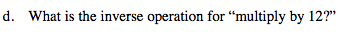If multiplication and division are inverse operations, how much do you have to divide by?